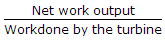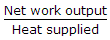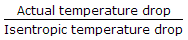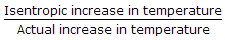# Mechanical Engineering - Thermodynamics

### Exercise :: Thermodynamics - Section 4

1.

Which of the following gas has the highest calorific value?

 A. Coal gas B. Producer gas C. Mond gas D. Blast furnace gas

Explanation:

No answer description available for this question. Let us discuss.

2.

The oxygen atom is __________ times heavier than the hydrogen atom.

 A. 2 B. 4 C. 8 D. 16

Explanation:

No answer description available for this question. Let us discuss.

3.

When the gas is cooled at constant pressure,

 A. its temperature increases but volume decreases B. its volume increases but temperature decreases C. both temperature and volume increases D. both temperature and volume decreases

Explanation:

No answer description available for this question. Let us discuss.

4.

The work ratio of a gas turbine plant is given by

 A.B.C.D.Explanation:

No answer description available for this question. Let us discuss.

5.

As per Charles' law, the volume of a given mass of a perfect gas varies __________ as its absolute temperature, when the absolute pressure remains constant.

 A. directly B. indirectly